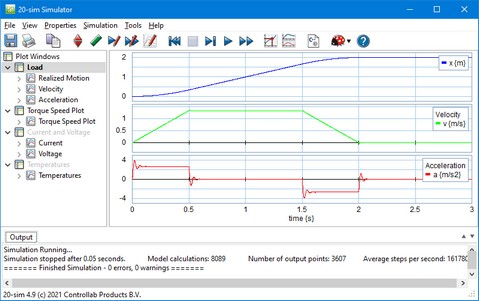﻿ 20-sim webhelp > Simulator > Running a Simulation > Plots

# Plots

The simulation plots are shown in the simulator tree at the left of the simulator window.You can open additional plots in 20-sim.

You can expand the simulator tree to see the plot windows. A plot window can contain one or more plots. A plot contains one or more curves. The curves show the simulated values of a chosen parameter.

Using the right mouse menu you can quickly:

 • Add or delete plot windows.
 • Add, delete, copy and paste plots.
 • Add, delete, copy and paste curves.
 • Change the appearance of a plot by selecting Plot Properties.
 • Change the variable by selecting Curve Properties.

Using the left mouse key you can:

 • Move plot windows and plot curves.
 • Copy plot windows and plot curves by clicking the Ctrl-key. A plus sign will appear next the the icon to show that you are going to copy.